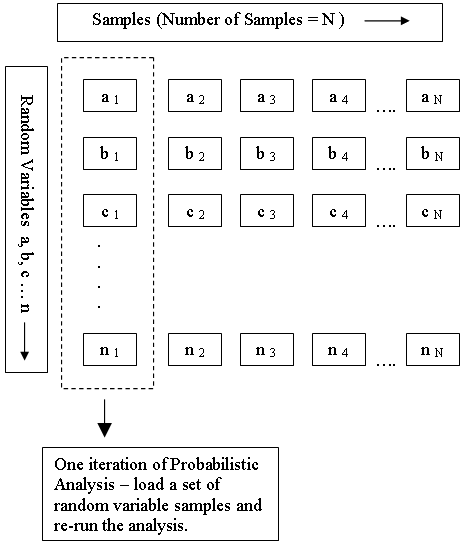The Rocscience International Conference 2021 Proceedings are now available. Read Now

Random Variables

In order to carry out a Probabilistic Analysis with UnWedge, you must define at least one input parameter as a random variable.

To define an input parameter as a random variable, select a statistical distribution for the variable (e.g., Normal, Uniform, etc.), and enter the required statistical parameters (e.g., mean, standard deviation, relative minimum, and maximum values).

The following input parameters can be defined as random variables in an UnWedge Probabilistic Analysis:

For information about the selection of statistical distributions, standard deviation and minimum/maximum values, see the Statistical Distributions topic.

How are the Random Variables used in the Analysis?

Each input parameter that is defined as a random variable, is sampled according to the statistical distribution you have defined for the variable, the Sampling Method and the Number of Samples (chosen in Project Settings). This generates N values of each random variable (where N = Number of Samples).

As shown in the following diagram, each iteration of the Probabilistic Analysis is carried out by loading a new set of random variable samples, and re-running the analysis. This is repeated N times where N = Number of Samples.

RANDOM VARIABLE SAMPLES USED IN PROBABILISTIC ANALYSISTo carry out a Probabilistic Analysis, at least one input parameter must be defined as random.